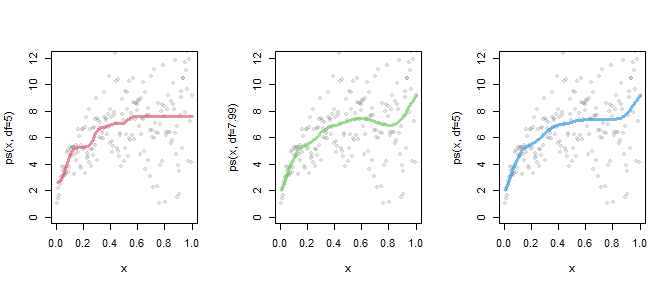# quantregGrowth: nonparametric quantile regression for growth charts and additive models

#### 2021-06-11

The package quantregGrowth aims to estimate the smooth, but unspecified, effect of numerical covariate(s) on one or more quantiles of the numerical response variable. The quantile regression equation can also include linear effect(s) of numerical or categorical covariates. Each flexible and unspecified relationship is expressed via a B-spline basis whose coefficients are somehow shrunken to control the wiggliness of the curve(s). The amount of smoothness is estimated as part of the model fitting as described in Muggeo et al., 2020.

The package relies on the well known quantreg package by Roger Koenker by exploiting full functionality and efficiency of its fitter functions.

## Growth charts (for the eager :-))

When focus is on multiple response quantiles (1%, 5%,…, 95%, say) depending on a single variable, the resulting fitted quantile curves are also referred as growth charts. Growth charts are smooth quantile trajectories at different probability values, ‘tau’, with the noncrossing property, namely the quantile curve at any specified tau value has to be always higher then the quantile curve at any lower probability value. Growth charts are used to build reference values of an outcome of interest with respect to another variable, usually age or time. Typical examples include height (or weight) or vocabulary size development versus the children’s age as investigated in wordbank website.

quantregGrowth uses B-splines with a penalty on the coefficients and relevant smoothing parameter selected’ within the estimation algorithm, and imposes proper constraints to prevent quantile crossing.

We build growth charts of height versus age using the data set SiChildren shipped with the package. The main function is gcrq() which requires the usual model formula inclduing the ps() function on the right hand side. gcrq() returns quantile curves at (default) probability values c(.1, .25, .5, .75, .9), but in this example we choose different values via the argument tau, and display the result by means of the plot method function:

library(quantregGrowth)
data(SiChildren)

o <-gcrq(height ~ ps(age), tau=seq(.05,.95,l=7), data=SiChildren)

plot(o, res=TRUE) #res=TRUE displays data too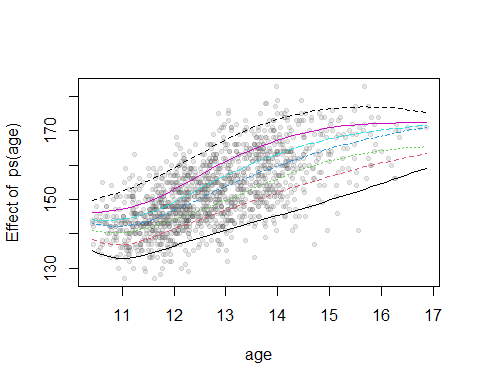In addition to the graphical output, we could look at the estimated quantile values can be obtained via the function charts(), which is a simple wrapper of predict.gcrq() to facilitate production of growth charts based on the fitted model. We display the quantiles just at some age values

charts(o, k=c(10,10.5,11,16,17)) #the quantile at the specified k values
>            0.05    0.2   0.35    0.5   0.65    0.8   0.95
> age 10   135.24 138.35 141.05 143.00 144.23 146.29 149.83
> age 10.5 133.02 136.86 140.29 142.25 144.00 146.85 151.83
> age 11   133.41 137.75 141.17 143.14 145.24 148.52 154.43
> age 16   154.36 160.47 164.16 168.39 170.16 172.18 176.94
> age 17   159.20 163.33 165.38 171.00 171.64 172.47 175.38

Results look reasonable, but there are some annoying features which need to be stressed: at around age=16 the highest quantile curve (the 95th) decreases, while some quantile curves (the 5th to the 65th) drop at early ages around 11 years and then increases again afterwards.

Broadly speaking, non monotonic growth charts could be biologically sound (for instance when modelling the body mass index), but in this example they are not. Likely, the sparseness of data on the left and right tails of age distribution, causes the drops in the fitted curves. Hence we re-fit the model by imposing positive monotonicity contraints via the argument monotone=1 in ps(),

oM<-gcrq(height ~ ps(age, monotone=1), data=SiChildren, tau=seq(.05,.95,l=7))

In the following, we report two plots: the first one focuses on the early ages by stressing differences between the fitted curves, and the second figure could be possibly closer to the usual growth charts, with a legend (set at the age axis value overlap=15), an underlying grid (with 15 vertical and 10 horizontal lines) and customized’ axis labels.

par(mfrow=c(1,2))
#the 1st plot..
plot(o, xlim=c(10.2,12.5), col=1, lty=3, lwd=2)
#the 2nd..
plot(oM, legend=TRUE, overlap=15, grid=list(x=15,y=10), col=2, lty=1,
ylab="Height (cm)", xlab="Age (years)")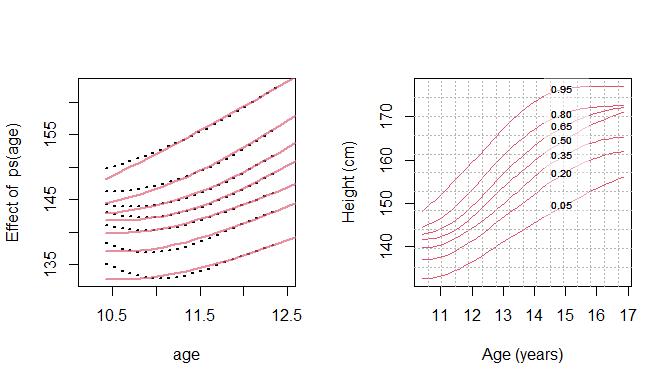Several options are available when plotting a gcrq object, see ?plot.gcrq.

quantregGrowth can deal with multiple smooth terms straightforwardly. We use data simulated via mgcv::gamSim function (example 1). We are interested in modelling nonparametrically the effect of four covariates on the median, say. At this aim, we call gcrq() with several ps() terms and a single tau value,

set.seed(1515)
d<-mgcv::gamSim(n=200, eg=1, verbose=FALSE) #verbose=TRUE suppresses the message..

o <- gcrq(y ~ ps(x0) + ps(x1) + ps(x2) + ps(x3), data=d, tau=.5)

The plots including the fitted relationships for all 4 terms are ready obtained by

# Plot the fit
par(mfrow=c(2,2))
plot(o, res=TRUE, col=2, conf.level=.95, shade=TRUE, cex.p=.6) #cex.p<1 to reduce the points..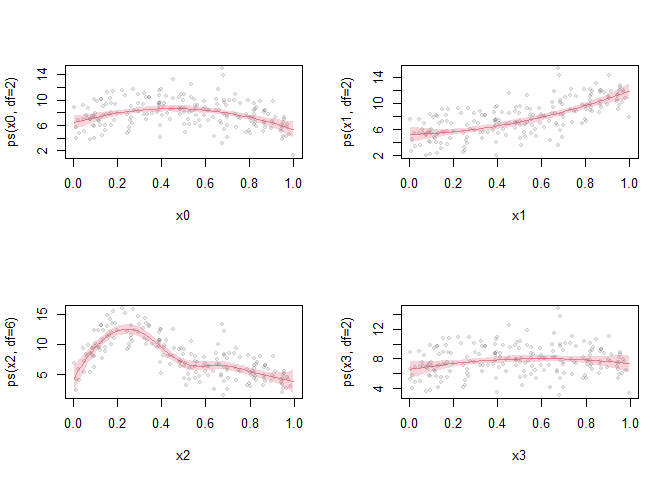where the y-label on each plot displays the edf of each smooth term. Note, when there are multiple covariates, res=TRUE portrays the partial residuals (and not the observed values).

## Additive model with linear terms

gcrq() can also include standard linear terms: for instance, the above plots suggest that a simple linear term would suffice to capture the relationships for x1 and x3. Therefore in the next model formula we include these variables outside the ps() function. We also display the model output via summary.gcrq().

o1<-gcrq(y ~ ps(x0) + x1 + ps(x2) + x3, data=d, tau=.5)

#the summary method
summary(o1)
> Call:
> gcrq(formula = y ~ ps(x0) + x1 + ps(x2) + x3, tau = 0.5, data = d)
>
> --------  Percentile: 0.50   check function:  157.69 -----
>
> parametric terms:
>                Est  StErr  |z| p-value
> (Intercept) 3.9769 0.6510 6.11   1e-09 ***
> x1          6.7935 0.8178 8.31  <2e-16 ***
> x3          0.6163 0.8100 0.76   0.447
> ---
> Signif. codes:  0 '***' 0.001 '**' 0.01 '*' 0.05 '.' 0.1 ' ' 1
>
> smooth terms:
>          edf
> ps(x0) 2.000
> ps(x2) 4.999
> =========================
>
> No. of obs = 200    No. of params = 25 (25 for each quantile)
> Overall check = 157.69  SIC = -0.105 on edf = 10 (ncross constr: FALSE)

For the parametric terms, the summary method returns point estimates, standard errors based on the sandwich formula, and $$p$$-values coming from the Wald statistic. Degrees of freedom for the smooth terms are printed next, along with some useful information of the fit.

Of course we could estimate the same ‘semiparametric’ model at several probability values

o2<-gcrq(y ~ x1 + x3 + ps(x0) + ps(x2), data=d, tau=c(.12,.25,.5,.7,.8,.9))

with the noncrossing constraints. Summary and plots can be obtained straightforwardly.

## Simple linear models

gcrq() can fit purely linear models, i.e. with no ps() term in the formula. Here a simple example.

n=100
x<-1:n/n
y<-2*x+rnorm(n)*rev(x)

o3 <-gcrq(y ~ x, tau=seq(.05,.95,l=10)) #fit 10 (noncrossing) regression quantiles

By means of plot.gcrq() with proper arguments, we display in the first plot the linear growth charts, and in the 2nd and 3rd we portray the tau-varying coefficients by setting axis.tau=TRUE,

par(mfrow=c(1,3))
plot(x,y)
plot(o3, term=1, axis.tau=TRUE)
plot(o3, term="x", axis.tau=TRUE, conf.level = .95, col=2)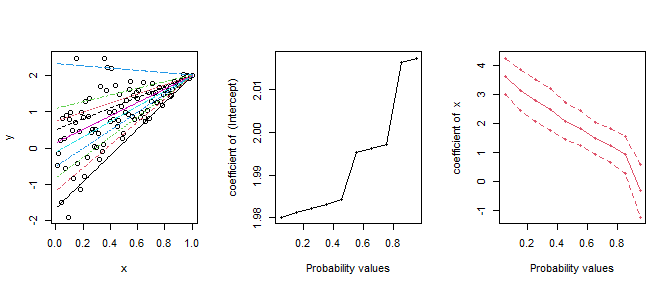The 2nd and 3rd plots could be useful to assess if and how the specified coefficient changes across the quantile curves.

## Customizing the penalty

A possibly useful feature of quantregGrowth is supplying a user-defined (multiplicative) penalty via the argument pen.matrix in ps(). The penalty matrix $$A$$, say, should be a matrix such that $$\lambda||A\beta||_1$$ is the penalization in the objective to be minimized. $$\beta$$ is the vector of spline coefficients and $$\lambda$$ is the smoothing parameter fixed or to be estimated. The example below illustrates how a proper penalty matrix can be set to force a zero slope in the fitted curve at large values of the covariate. At this aim we consider weighted first-order differences by assigning a very large weight to the rightmost differences to fulfill the zero slope constraint. Overall, the penalty is $$\sum_j^{J-1} |\beta_j-\beta_{j-1}|w_j=||diag(w) D^{(1)}\beta||_1= ||A\beta||_1$$, where $$D^{(1)}$$ is the first-order differences matrix and $$w$$ is the weight vector as defined below. To build the customized penalty matrix with appropriate dimension, it is probably helpful to set explicitly the number of the basis coefficients via the arguments ndx (number of covariate intervals which defaults to $$min(n/4,12)$$) and deg (spline degree which defaults to 3): Each basis has ndx+deg-1 identifiable coefficients.

data(growthData)

D1 <- diff(diag(23),dif=1)[,-1] #the 1st order diff matrix
w <- c(rep(1,15),rep(1000,7)) #the weight vector s.t. length(w)=nrow(D1)
A <- diag(w) %*% D1 #the penalty matrix

o4 <-gcrq(y~ps(x, ndx=20, pen.matrix=A), data=growthData, tau=.5)

The length of zero slope depends on number of large values in $$w$$, in the above example rep(100,7). Increasing (decreasing) the number of large values would lead to increase (decrease) the zero slope interval.

Figure below reports results of the aforementioned constrained fit, along with the fits obtained using unweighted first order-differences without and with additional monotonicity constraints

o5 <-gcrq(y~ps(x, d=1), data=growthData, tau=.5)
o6 <-gcrq(y~ps(x, d=1, monotone = 1), data=growthData, tau=.5)

par(mfrow=c(1,3))
plot(o4, res=TRUE, col=2, lwd=3, ylim=c(0,12))
plot(o5, res=TRUE, col=3, lwd=3, ylim=c(0,12))
plot(o6, res=TRUE, col=4, lwd=3, ylim=c(0,12))